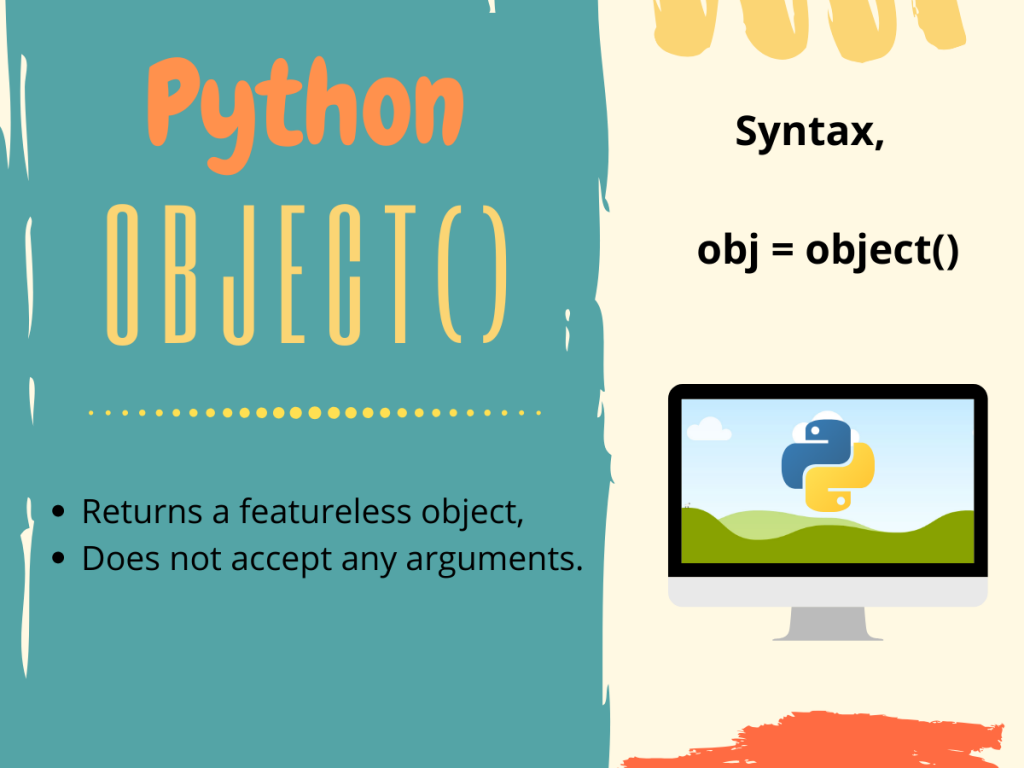# What is the Python object() Method?Welcome folks! for today in this tutorial, we are going to be discussing the Python object() method and class.

So let us get started with the basic definition and syntax of the Python `object()` method.

## The Python object() Method

The Python `object()` method, when called, returns a new featureless object of the `object` class. This method requires no arguments. The syntax for using the object() method is given below.

```obj = object()
```

Here, `obj` is now a featureless object which holds the built-in properties and methods which are the default for all classes.

Typically, the Python `object` class serves as the base class for all classes including user-defined ones. Hence all classes inherit from the Object class in Python. This is the default case for Python 3.x versions. But for Python 2.x versions for defining a new-style class, one needs to explicitly make the class inherit from the object class as shown below.

```class my_class(object):
...
```

## Python object() Method – Sample Program

Now that we have gathered a fair knowledge about the object class and `object()` method let us jump into an example to get a clearer picture.

```#object() object
obj = object()

print("obj = ", obj)
print("Type of obj = ", type(obj))
print(dir(obj)) #attributes of obj
```

Output:

```obj =  <object object at 0x01374040>
Type of obj =  <class 'object'>
['__class__', '__delattr__', '__dir__', '__doc__', '__eq__', '__format__', '__ge__', '__getattribute__', '__gt__', '__hash__', '__init__', '__init_subclass__', '__le__', '__lt__', '__ne__', '__new__', '__reduce__', '__reduce_ex__', '__repr__', '__setattr__', '__sizeof__', '__str__', '__subclasshook__']
```

Simply here we have created a new object `obj` using the `object()` method and determine its type as well as all of its attributes using type() and dir() methods respectively.

From the above output, we can clearly see that `obj` belongs to the object class. Note, obj does not have a `__dict__` attribute. Hence, we can’t assign arbitrary attributes to an instance of the `object` class.

## Properties of the Python object() Method

In this section we are going to look at some of the `object` properties, explore it’s uses and try to play around with it.

```class demo():
a = 10
b = 20

#declaring object of user-defined class
d1 = demo()

#featureless objects
d2 = object()
d3 = object()

print("d2 == d3? ", d2 == d3)
print("issubclass(demo, object)? ", issubclass(demo, object)) #demo inherites from object class
print("isinstance(d1, object)? ", isinstance(d1, object))
print("Type of d2 = ", type(d2))
print(d2)
```

Output:

In the code above, `demo` is a user-defined class with some values a and b. `d1` is an object from the demo class. Whereas, `d2` and `d3` are empty objects from the object class created using the `object()` method.

It is clear from the above output that even d2 and d3 are two empty objects of the same type, `d2==d3` condition is not satisfied. Again using both the issubclass() and isinstance() methods over `demo` class and `d1` object respectively, we can infer the following statements.

1. the `demo` class is a subclass of the `object` class hence inherits from it,
2. And, d1(demo object) is also an instance of the `object` class(base class).

You can also see that we cannot directly print an empty object, so we get `<object object at 0x007C4040>`.

## Summing Up

That’s it for this topic. For more information we highly recomend going through the links below in the reference seection.

For any further questions related to this topic, feel free to use the comments below.

Happy learning.